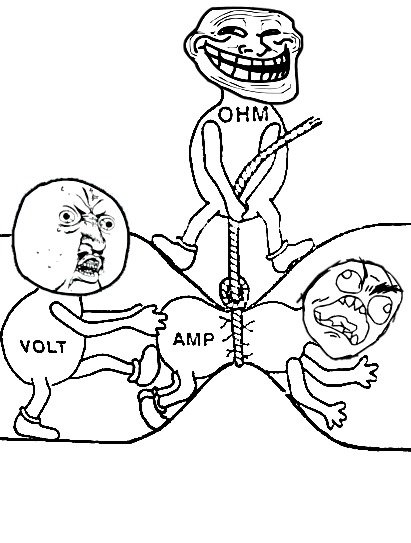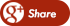﻿ RELATION BETWEEN VOLTAGE AND CURRENTBack

## RELATION BETWEEN VOLTAGE AND CURRENT

By Admin onWHAT IS THE RELATION BETWEEN VOLTAGE AND CURRENT?

Ohm’s Law explains the relationship between voltage (V or E), current (I) and resistance (R) . Used by electricians, automotive technicians, stereo installers.

VOLTAGE  (V)

It is the push or pressure behind current flow through a circuit, and is measured in (V) volts.

CURRENT

Current refers to the quantity/volume of electrical flow. Measured in Amps (A).

RESISTANCEResistance to the flow of the   current. Measured in Ohms

1.  Assuming the resistance does not change:

As voltage increases, current increases.

As voltage decreases, current decreases.

2.  Assuming the voltage does not change:

As resistance increases, current decreases.

As resistance decreases, current increases.

Resistors

To decrease the amount of voltage applied to a component .The value of the resistor is marked on the body using coloured rings.Voltage, also called electromotive force, is a quantitative expression of the potential difference in charge between two points in an electrical field.

The greater the voltage, the greater the flow of electrical current (that is, the quantity of charge carriers that pass a fixed point per unit of time) through a conducting or semiconducting medium for a given resistance to the flow. Voltage is symbolized by an uppercase italic letter V or E. The standard unit is the volt, symbolized by a non-italic uppercase letter V. One volt will drive one columb (6.24 x 1018) charge carriers, such as electrons, through a resistance of one ohm in one second.